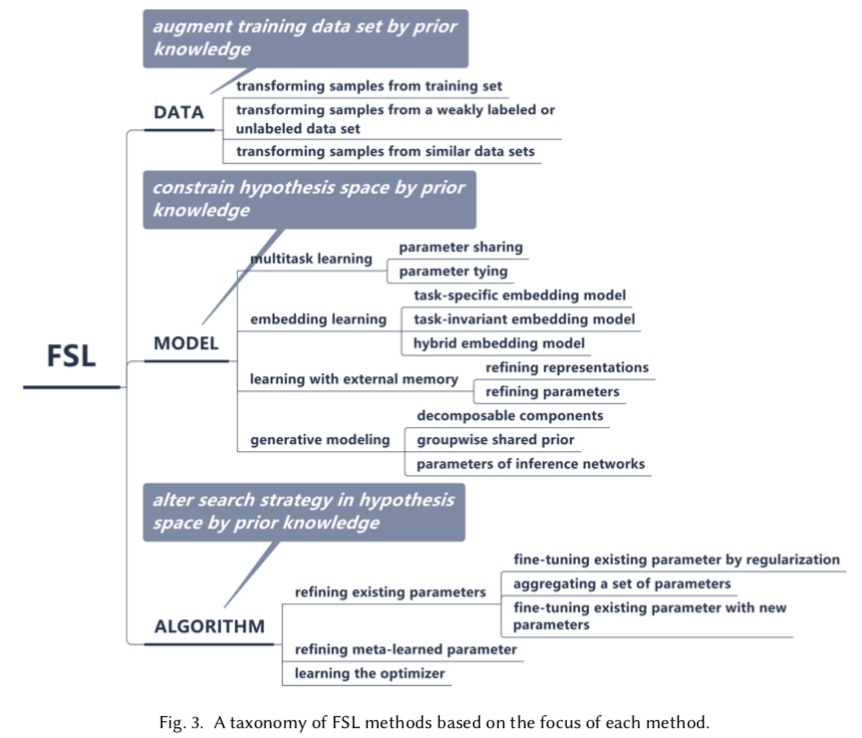# A Survey on Few-shot Learning | Introduction and Overview

## 当前最新小样本学习综述

Posted by JoselynZhao on April 17, 2020

Author list YAQING WANG, Hong Kong University of Science and Technology and Baidu Research QUANMING YAO∗, 4Paradigm Inc. JAMES T. KWOK, Hong Kong University of Science and Technology LIONEL M. NI, Hong Kong University of Science and Technology

• （i）数据，它使用先验知识来增强监督经验；
• （ii）模型，它使用先验知识来减小假设空间的大小；
• （iii）算法，该算法使用先验知识来改变对给定假设空间中最佳假设的搜索。

@[toc] Additional Key Words and Phrases: Few-Shot Learning, One-Shot Learning, Low-Shot Learning, Small Sample Learning, Meta-Learning, Prior Knowledge

# 01 Introduction

current AI techniques cannot rapidly generalize from a few examples. The aforementioned successful AI applications rely on learning from large-scale data.

In contrast, humans are capable of learning new tasks rapidly by utilizing what they learned in the past. examples:

a child who learned how to add can rapidly transfer his knowledge to learn multiplication given a few examples (e.g., 2 × 3 = 2 + 2 + 2 and 1 × 3 = 1 + 1 + 1). Another example is that given a few photos of a stranger, a child can easily identify the same person from a large number of photos.

### Bridging this gap between AI and humans is an important direction.

In order to learn from a limited number of examples with supervised information, a new machine learning paradigm called Few-Shot Learning (FSL) [35, 36] is proposed.

### FSL can also help relieve the burden of collecting large-scale supervised data.

although ResNet  outperforms humans on ImageNet, each class needs to have sufficient labeled images which can be laborious to collect.

Examples include

• image classification 
• image retrieval 
• object tracking 
• gesture recognition 
• image captioning
• video event detection 
• language modeling 
• neural architecture search 

### Driven by the academic goal for AI to approach humans and the industrial demand for inexpensive learning, FSL has drawn much recent attention and is now a hot topic.

Many related machine learning approaches have been proposed：

• meta-learning [37, 106, 114],
• embedding learning [14, 126, 138]
• generative modeling [34, 35, 113].

### Contributions of this survey can be summarized as follows

• 我们给出了FSL的正式定义，该定义自然与[92，94]中的经典机器学习定义相关。该定义不仅足够笼统地包括现有的FSL作品​​，而且足够具体以阐明FSL的目标是什么以及我们如何解决它。该定义有助于在FSL领域设定未来的研究目标。
• 我们通过具体示例列出了FSL的相关学习问题，阐明了它们与FSL的相关性和差异。这些讨论可以帮助更好地区分FSL，并将其定位在各种学习问题之间。
• 我们指出，FSL监督学习问题的核心问题是不可靠的经验风险最小化器，它是基于机器学习中的错误分解进行分析的。这为以更组织和系统的方式改进FSL方法提供了见识。
• 我们进行了广泛的文献回顾，并从数据，模型和算法的角度将它们整理成统一的分类法。我们还提供了一些见解的摘要，并就每种类别的利弊进行了讨论。这些可以帮助您更好地理解FSL方法。
• 我们在问题设置，技术，应用和理论方面为FSL提出了有希望的未来方向。这些见解基于FSL当前发展的弱点，并可能在将来进行改进。

## 01. 2 Notation and Terminology

Consider a learning task $$T$$, FSL deals with a data set $$D = \left\{D_{train},D_{test}\right\}$$ consisting of a training set $$D_{train} = \left\{(x_i,y_i)\right\}_{i=1}^I$$ whereI $$I$$ is small,and a testing set $$D_{test} = \left\{x_{test}\right\}$$. Letp(x,y) be the ground-truth joint probability distribution(联合概率分布) of input x and output y, and $$\hat{y}$$ be the optimal hypothesis from x to y. FSL learns to discover $$\hat{y}$$ by fitting $$D_{trian}$$ and testing on $$D_{test}$$. θ denotes all the parameters used by h.

A FSL algorithm is an optimization strategy that searches H in order to find the θ that parameterizes the best h*. The FSL performance is measured by a loss function $$l(\hat{y},y)$$ defined over the prediction $$\hat{y}= h(x;θ)$$ and the observed output y.

# 02 Overview

## 02.1 Problem definition

### how machine learning is defined

A computer program is said to learn from experience E with respect to some classes of task T and performance measure P if its performance can improve with E on T measured by P . examples:

consider an image classification task (T ), a machine learning program can improve its classification accuracy (P) through E obtained by training on a large number of labeled images (e.g., the ImageNet data set ).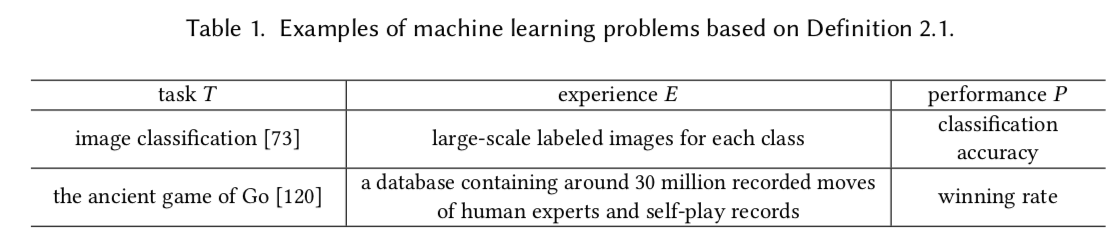FSL is a special case of machine learning, which targets at obtaining good learning performance given limited supervised information provided in the training set $$D_{train}$$.

### The definition of FSL

Few-Shot Learning (FSL) is a type of machine learning problems (specified by E, T and P), where E contains only a limited number of examples with supervised information for the target T .

few-shot classification learns classifiers given only a few labeled examples of each class. example applications:

• image classification 
• sentiment classification(情绪分类) from short text 
• object recognition .

### N-way-K-shot classification[37,138]

$$D_{train}$$ contains I = KN examples from N classes each with K examples.

### Few-shot regression [37, 156]

estimates a regression function h given only a few input-output example pairs sampled from that function, where output $$y_i$$ is the observed value of the dependent variable y, and $$x_i$$ is the input which records the observed value of the independent variable x.

### few-shot reinforcement learning [3, 33]

targets at finding a policy given only a few trajectories consisting of state-action pairs.

### three typical scenarios of FSL

• 像人类一样充当学习的试验床。为了迈向人类智能，计算机程序能够解决FSL问题至关重要。一个流行的任务（T）是仅给出几个例子就生成一个新角色的样本。受人类学习方式的启发，计算机程序使用E进行学习，E由既有监督信息的给定示例以及受过训练的概念（如零件和关系）作为先验知识组成。通过视觉图灵测试（P）的通过率评估生成的字符，该测试可区分图像是由人还是由机器生成的。有了这些先验知识，计算机程序还可以学习分类，解析和生成新 -的手写字符，例如人类。
• 学习罕见的情况。当很难或不可能获得带有监督信息的足够示例时，FSL可以为罕见情况学习模型。例如，考虑一个药物发现任务（T），该任务试图预测一个新分子是否具有毒性作用。通过新分子的有限测定和许多类似分子的测定（既有知识）获得的E，正确分配为有毒或无毒（P）分子的百分比会提高。
• 减少数据收集工作量和计算成本。 FSL可以帮助减轻收集大量带有监督信息的示例的负担。考虑少量拍摄图像分类任务（T）。图像分类精度（P）随目标类别T的每个类别的少量标记图像获得的E以及从其他类别中提取的先验知识（例如原始图像到共同训练）而提高。成功完成此任务的方法通常具有较高的通用性。因此，它们可以轻松地应用于许多样本的任务。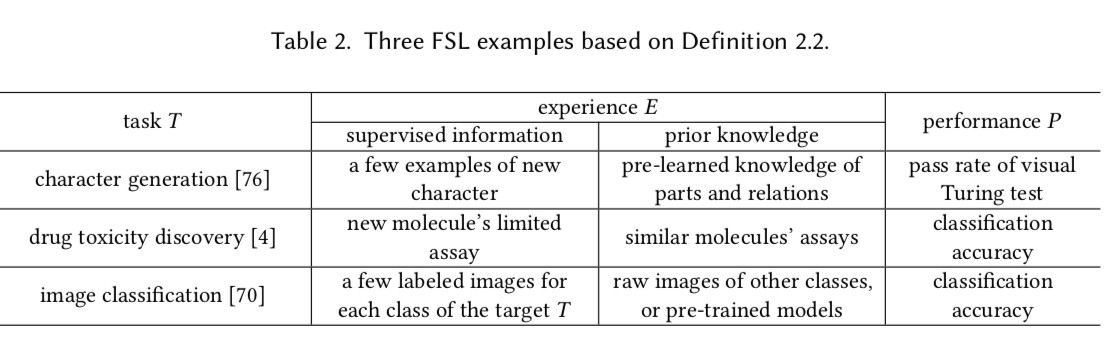One typical type of FSL methods is Bayesian learning [35, 76]. It combines the provided training set $$D_{train}$$ with some prior probability distribution which is available before $$D_{train}$$ is given .

When there is only one example with supervised information in E,FSL is called one-shot learning [14, 35, 138]. When E does not contain any example with supervised information for the target T , FSL becomes a zero-shot learning problem (ZSL) .

ZSL requires E to contain information from other modalities(形式) (such as attributes, WordNet, and word embeddings used in rare object recognition tasks), so as to transfer some supervised information and make learning possible.

## 02.2 Relevant Learning Problems

### Weakly supervised learning 

only a small amount of samples have supervised information.

this can be further classified into the following:

• Semi-supervised learning , which learns from a small number of labeled samples and (usually a large number of) unlabeled samples in E. Positive-unlabeled learning  is a special case of semi-supervised learning, in which only positive and unlabeled samples are given.
• Active learning , which selects informative unlabeled data to query an oracle for output y. This is usually used for applications where annotation labels are costly, such as pedestrian detection.

weakly supervised learning with incomplete supervision mainly uses unlabeled data as ad- ditional information in E, while FSL leverages various kinds of prior knowledge such as pre-trained models, supervised data from other domains or modalities and does not restrict to using unlabeled data. Therefore, FSL becomes weakly supervised learning problem only when prior knowledge is unlabeled data and the task is classification or regression.

### Transfer learning 

It can be used in applications such as cross-domain recommendation, WiFi localization across time periods, space and mobile devices. Domain adaptation  is a type of transfer learning in which the source/target tasks are the same but the source/target domains are different. example：

in sentiment analysis, the source domain data contains customer comments on movies, while the target domain data contains customer comments on daily goods.

### Meta-learning 

Meta-learning  improves P of the new task T by the provided data set and the meta- knowledge extracted across tasks by a meta-learner. Specifically, the meta-learner gradually learns generic information (meta-knowledge) across tasks, and the learner generalizes the meta-learner for a new task T using task-specific information.

the meta-learner is taken as prior knowledge to guide each specific FSL task.

## 02.3 Core Issue

we illustrate the core issue of FSL based on error decomposition（分解） in supervised machine learning [17, 18]

### 02.3.1 Empirical Risk Minimization.

Given a hypothesis h, we want to minimize its expected risk R, which is the loss measured with respect to p(x,y). Specifically,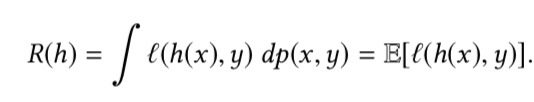因为p(x,y)是未知的， the empirical risk（训练集$$D_{train}$$的I个样本的平均loss）表达如下：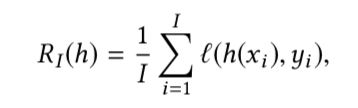The empirical risk 通常被用作$$R(h)$$的proxy，可以使得empirical risk minimization.(可能有一些正则化)

• data
• model， which determines H
• algorithm，which 搜索满足data的最优$$h_I$$

### 02.3.2 Unreliable Empirical Risk Minimizer.

estimation error 可以通过增加样本量来减少[17,18,41]。所以，当有充足的训练监督信息数据的时候，estimation error 很小。

this is the core issue of FSL supervised learning： the empirical risk minimizer $$h_I$$ is no longer reliable.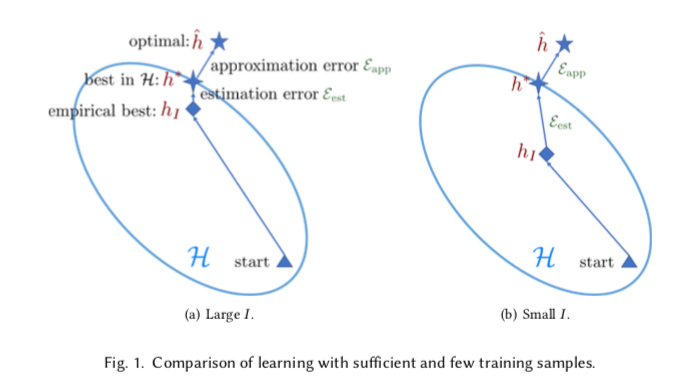## 02.4 Taxonomy

• Data. 这种方法是利用先验知识来增强训练集，扩大I的数量。 使得在这种情况下，标准的机器学习模型和算法可以被使用。
• Model。 这种方式是利用先验知识来约束假设空间的复杂性，使得假设空间变小。在这种情况下，训练集足够去学习一个可靠的$$h_I$$.
• Algorithm. 这种方法使用先验知识来搜索$$\theta$$,$$\theta$$ 参数化最佳的h。 先验知识通过提供良好的初始化（图2（c）中的灰色三角形）或指导搜索步骤（图2（b）中的灰色虚线）来更改搜索策略。 对于后者，最终的搜索步骤受先验知识和经验风险最小化因素的影响。